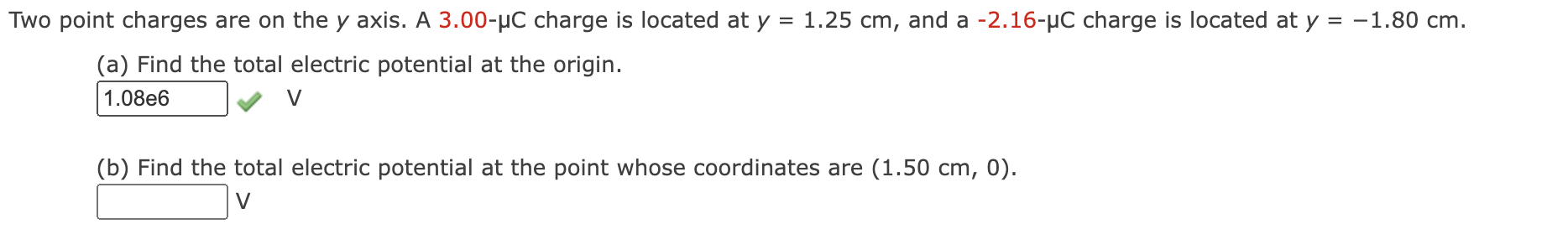Home / Expert Answers / Physics / two-point-charges-are-on-the-y-axis-a-3-00-mu-mathrm-c-charge-is-located-at-y-1-pa164

# (Solved): Two point charges are on the $$y$$ axis. A $$3.00-\mu \mathrm{C}$$ charge is located at $$y=1 ...Two point charges are on the \( y$$ axis. A $$3.00-\mu \mathrm{C}$$ charge is located at $$y=1.25 \mathrm{~cm}$$, and a $$-2.16-\mu \mathrm{C}$$ charge is located at $$y=-1.80$$ cm. (a) Find the total electric potential at the origin. $$\mathrm{V}$$ (b) Find the total electric potential at the point whose coordinates are $$(1.50 \mathrm{~cm}, 0)$$.

We have an Answer from Expert